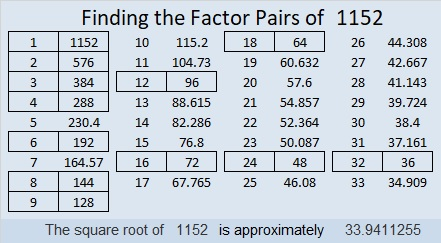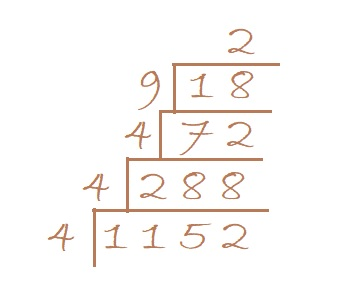# 1152 Will You See All the Prime Factors in This Factor Tree?

Will you see all the prime factors in this factor tree when all of the factors are the same color? You know how to count, but would you possibly not count one of the prime factors or possibly count one of them twice?Is it easier to count the prime factors in the following factor trees?If you happen to have two different colors of ink and/or pencils around when you make factor trees, they might be easier to read especially if the factored number has a lot of factors like 1152 does.

Here’s what I’ve learned about the number 1152:

• 1152 is a composite number.
• Prime factorization: 1152 = 2 × 2 × 2 × 2 × 2 × 2 × 2 × 3 × 3, which can be written 1152 = 2⁷ × 3²
• The exponents in the prime factorization are 7 and 2. Adding one to each and multiplying we get (7 + 1)(2 + 1) = 8 × 3 = 24. Therefore 1152 has exactly 24 factors.
• Factors of 1152: 1, 2, 3, 4, 6, 8, 9, 12, 16, 18, 24, 32, 36, 48, 64, 72, 96, 128, 144, 192, 288, 384, 576, 1152
• Factor pairs: 1152 = 1 × 1152, 2 × 576, 3 × 384, 4 × 288, 6 × 192, 8 × 144, 9 × 128, 12 × 96, 16 × 72, 18 × 64, 24 × 48, or 32 × 36
• Taking the factor pair with the largest square number factor, we get √1152 = (√576)(√2) = 24√2 ≈ 33.94112The last two digits of 1152 are 52, a number divisible by 4, so 1152 can be evenly divided by 4.
1 + 1 + 5 + 2 = 9, so 1152 is divisible by 9.

It is so easy to tell if a number can be evenly divided by 4 or 9 AND it is so easy to divide by 4 or by 9. When I make a factor cake, I like to see if the current layer of the cake is divisible by 4 or by 9 before I check to see if it is divisible by a prime number.From that cake, I can quickly tell that 1152 = 2⁷ × 3² by simply counting by 2’s to find the powers of 2 and 3. All the numbers being the same color doesn’t even slow me down.

I can also easily find the √1152 by taking the square root of everything on the outside of the cake:
√1152  = √(4·4) · (√4)(√9)(√2) = (4·2·3)√2 = 24√2

Since MOST square roots that can be simplified are divisible by 4, or by 9, or by both, this is a good strategy to find their square roots.

1152 is the sum of the fourteen prime numbers from 53 to 109,
and it is the sum of the twelve prime numbers from 71 to 127.

34² – 2² = 1152 so we are only 2² = 4 numbers away from the next perfect square.

1152 looks interesting when it is written in these bases:
It’s 800 in BASE 12 because 8(12²) = 1152,
242 in BASE 23 because 2(23²) + 4(23) + 2(1) = 1152
200 in BASE 24 because 2(24²) = 1152,
WW in BASE 35 (W is 32 base 10) because 32(35) + 32(1) = 32(36) = 1152, and
it’s W0 in BASE 36 because 32(36) = 1152

# 6 A Piece of Cake

### Birthday Puzzles for My Daughter:

Happy birthday, Kathy! I hope your day is wonderful. You have grown into a beautiful, talented, prayerful, intelligent, hard-working, and loving young woman.  I am grateful you are my daughter.  So for your birthday today and for this blog, I’ve created three special puzzles: the first is a birthday cake to celebrate your happy day. To highlight your love of music, the second puzzle is a quarter note. The third puzzle is either a violin, a guitar, or a ukulele, you decide. I love listening as you sing or as you play any of those instruments or the piano. Today for your birthday I will also cut down a tree and make yet another cake with two birthday candles on top in this blog post.  So have a fun birthday, today.  I love you.Click 12 Factors 2013-11-14 for more puzzles.### Factor Trees vs. Factor Cakes:

What did I mean by cutting down a tree and making yet another cake? Today I will discuss two methods for finding the prime factors of a whole number. One method is making a factor tree and the other is the cake method. To factor a number means to write it as the product of two or more factors. When those two or more factors are all prime factors, it is called a prime factorization of the number. A composite number always has more than two factors. A prime number always has exactly 2 factors, 1 and itself. (ZERO and ONE are neither prime or composite numbers.) Usually, to find the prime factors of a number, a person will usually make a factor tree. The following example shows how this is done:From this example, you can certainly understand why this algorithm is called a factor tree.  It looks exactly like a perfectly-shaped evergreen tree.  The problem is that a factor tree doesn’t always look so neat and trim.  Here is a factor tree that even Charlie Brown wouldn’t choose:720 isn’t even that big of a number, but gathering all of the prime numbers from the factor tree and putting them in numerical order would be like picking up a bunch of scattered leaves. It would be like doing . . . yard work.  Imagine if you had a number that had many more factors. If one or two of the factors gets lost in the mess, your answer wouldn’t be correct. Notice that some of the prime factors of 720 (2,2,2,2,3,3,5) are not as easy to see as others on the factor tree.  That is why I want to chop down that tree. Even if you like to do yard work, do you really want to deal with that big of a mess, . . . especially when you can have cake instead?  Look, the cake method is so much more pleasing to the eye, and it is simply an extension of the very familiar division algorithm:With the cake method, the more factors you have, the bigger the cake will be, but it will always be neatly organized with all the factors on the outside of the cake.  And if the largest prime factor of your given number is eleven, you will also have two candles on top of your cake!  I find using the cake method to be much less confusing than using a factor tree.  Yes, finding prime factors can actually be a piece of cake. The only disadvantage to the cake method is that since you work from the bottom up you have to leave enough space for the cake to rise.

Still, in spite of my opinion, it is best to use whichever method you are more comfortable with.

Now if your appetite for cake has not been satisfied, click on one of the links below for a nice variety of cakes shared by other bloggers.

### Factors of the Number 6:6 is a composite number. 6 = 1 x 6 or 2 x 3. Factors of 6: 1, 2, 3, 6. Prime factorization: 2 x 3.When 6 is a clue in the FIND THE FACTORS  puzzle, the pair that will work for that particular puzzle might be 1 x 6, or it might be 2 x 3.

### A Sum-Difference Puzzle Featuring the Number 6 and its Factors:

Look at the factor pair puzzle above. Perhaps you will notice that
2 + 3 = 5 and 6 – 1 = 5.
Those are the facts you need to complete the Sum-Difference puzzle below.